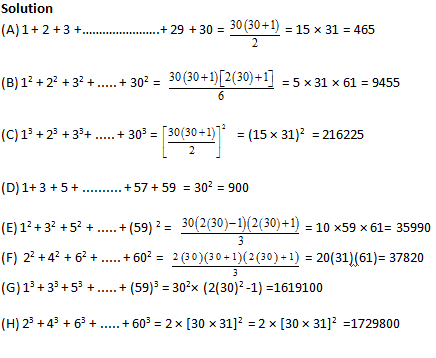Properties of Addition of Natural Numbers:
Sum of 1st ‘n’ Natural numbers =  1 + 2 + 3 + …. + n = $\frac{n\left( n+1 \right)}{2}$

Sum of squares of 1st ‘n’ natural number  =  12 + 22 + 32 + ….. + n2  = $\frac{n\left( n+1 \right)\left( 2n+1 \right)}{6}$

Sum of cubes of 1st ‘n’ Natural number  =  13 + 23 + 33 + ….. + n3  = ${{\left( \frac{n\left( n+1 \right)}{2} \right)}^{2}}$

Sum of 1st ‘n’ Odd natural number  =  1+ 3 + 5 + ….. + (2n – 1)  = n2

Sum of 1st ‘n’ even natural numbers  = 2 + 4 + 6+ …….2n = n (n+1)

Sum of squares of 1st ‘n’ Odd natural number = 12 + 32 + 52 + ….. + (2n – 1) 2 = $\frac{n\left( 2n-1 \right)\left( 2n+1 \right)}{3}$

Sum of squares of 1st ‘n’ Even natural number =  22 + 42 + 62 + ….. + (2n)2 = $\frac{2n\left( n+1 \right)\left( 2n+1 \right)}{3}$

Sum of cubes of 1st  ‘n’ Odd natural number =  13 + 33 + 53 + ….. + (2n -1)3 = n2 (2n2 -1)

Sum of cubes of 1st  ‘n’ Even natural number  =  23 + 43 + 63 + ….. + (2n)3 = 2 [n (n + 1)]2

Some examples for the above properties:

Find the sum of:

(A) First 30 natural numbers

(B) Squares of first 30 natural numbers

(C) Cubes of first 30 natural numbers

(D) First 30 odd natural numbers

(E) Squares of first odd 30 natural numbers

(F) Squares of first even 30 natural numbers

(G) Cubes of first odd 30 natural numbers

(H) Cubes of first even 30 natural numbersGet Posts Like This Sent to your Email
Updates for Free Live sessions and offers are sent on mail. Don't worry: we do not send too many emails..:)
Get Posts Like This Sent to your Email
Updates for Free Live sessions and offers are sent on mail. Don't worry: we do not send too many emails..:)